• 多元线性回归预测模型
千次阅读
2022-05-11 21:41:20

使用多元线性回归对电力数据进行预测，其中时间、干球温度、露点温度、湿球温度、温度、电价为自变量、电力负荷为因变量.

 时间 干球温度 露点温度 湿球温度 温度 电价 电力负荷matlab 算法
更多相关内容
• 针对传统的多元线性回归预测方法处理时间长且受内存限制的特点，对时序样本数据设计了基于 MapReduce的并行多元线性回归预测模型。模型由三组MapReduce过程组成，分别求解由历史数据所构成叉积矩阵的特征向量和标准...
• 根据黄河北煤田赵官井田7号煤层小断层资料,选取断层走向延展长度、断层落差和断层倾角3个因素,运用多元回归分析方法,建立了7号煤层NE、NEE和NW向小断层延伸长度的预测模型。实际应用表明,该模型的预测精度较高,比较...
• //通过spark的MLib建立多元线性回归模型 //setFeaturesCol:通过别名指定哪些列是自变量列 //setLabelCol:指定因变量的列名 //setFitIntercept(true):表示计算截距项系数,截距项的系数可以不计算,但自变量的系数必须...

import org.apache.spark.SparkConf
import org.apache.spark.SparkContext
import org.apache.spark.sql.SQLContext
import org.apache.spark.ml.feature.VectorAssembler
import org.apache.spark.ml.regression.LinearRegression
object Driver01 {
def main(args: Array[String]): Unit = {

val conf=new SparkConf().setMaster("local").setAppName("lr")

val sc=new SparkContext(conf)

val sqc=new SQLContext(sc)//创建sparksql上下文对象，用于创建或转化DataFrame

val data=sc.textFile("D://bigdata/data/ml/lritem.txt")

val r1=data.map{x=>
val info=x.split("\\|")
val y=info(0).toDouble
val x1=info(1).split(" ")(0).toDouble
val x2=info(1).split(" ")(1).toDouble
(x1,x2,y)
}

//为了满足建模需求，RDD[(x1,x2,y)]->DataFrame(x1,x2,y) 数据表模型
//RDD转化成DataFrame的需求:1)RDD中每个元素必须是元组类型 2)DataFrame的列的个数以及顺序要和元组一致
val df1=sqc.createDataFrame(r1).toDF("x1","x2","y")

//VectorAssembler向量转化工具
//setInputCols:指定自变量的列名
//setOutputCol:自变量的别名,后续建模时通过别名找到所有自变量列
val vectorAss=new VectorAssembler().setInputCols(Array("x1","x2"))
.setOutputCol("features")

val df1Vectors=vectorAss.transform(df1)

//通过spark的MLib建立多元线性回归模型
//setFeaturesCol:通过别名指定哪些列是自变量列
//setLabelCol:指定因变量的列名
//setFitIntercept(true):表示计算截距项系数,截距项的系数可以不计算,但自变量的系数必须计算
//fit:带入数据建模
val model=new LinearRegression().setFeaturesCol("features")
.setLabelCol("y")
.setFitIntercept(true)
.fit(df1Vectors)

val coef=model.coefficients//获取自变量系数值
val intercept=model.intercept//获取截距项系数值

val predictReasult=model.transform(df1Vectors)
predictReasult.show()

//可以通过模型进行预测
val testRDD=sc.makeRDD(List((10,500,0)))
val testDF=sqc.createDataFrame(testRDD).toDF("x1","x2","y")
val testDFVectors=vectorAss.transform(testDF)
val testPredictResult=model.transform(testDFVectors)
testPredictResult.show()

//模型检验
//获取模型的多元R2值，这个指标可以评估模型拟合的优良性。
//最大值为1，越趋近1，表明模型对数据的拟合越好
//在生产环境下，R2在0.55以上都可以接受的
val R2=model.summary.r2
println(R2)
}
}

展开全文spark 机器学习
• 利用多元线性回归和BP神经网络理论,分别对矿井瓦斯涌出量进行了预测,最后建立了多元线性回归与BP神经网络的组合预测模型。该模型兼顾了多元回归分析的非线性特性和神经网络的时序特性,通过具体的实例研究,对比了各种...
• 目录：一、引言 二、理想场景的预设 三、多元线性回归模型 四、鸢尾花数据集

目录：

一、引言

二、理想场景的预设

三、多元线性回归模型

四、鸢尾花数据集

一、引言：

多元线性回归是一元线性回归的多维运用。在一元线性回归中，我们用自变量系数表示对自变量与因变量之间的解释因素。那么，在多元线性回归中，我们引入多个解释变量，对同一个个体在不同方面的解释进行多维度的衡量。

二、理想场景的预设：

为了便于理解，我们可以假设有一幢楼进行售卖，用y表示房子的房价，x表示这间房子的不同解释因素，如：面积、位置、采光度、装修等等（影响一间房子的价格绝不仅仅是面积的大小，因此这样的解释是合理的）。

那么，我们就要列举出一个能够综合评判房屋价格的一个数学模型，通过不同维度的计量，从而得到一个能够较全面衡量一间房屋综合价值的数值（类似于国民GDP）。

由此，我们便引入了多元线性回归模型，为了便于观察，我们还引入了基于矩阵运算的模型分析。

三、多元线性回归模型

模型引入：

通常情况下，一般的多元回归模型写法很多，例如：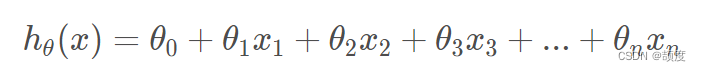这样写太过于繁杂，并且在实际求解的过程中并不能简化我们的运算。为此，引入矩阵运算。

假定x的矩阵为：X=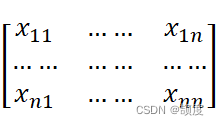，对应的θ的矩阵为：θ=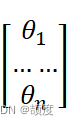在X中添加一个列向量1，得到：X=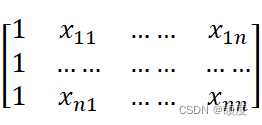通过矩阵运算，可以将hθ（x）=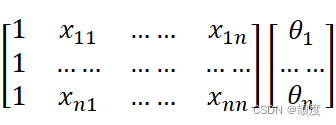推导损失函数：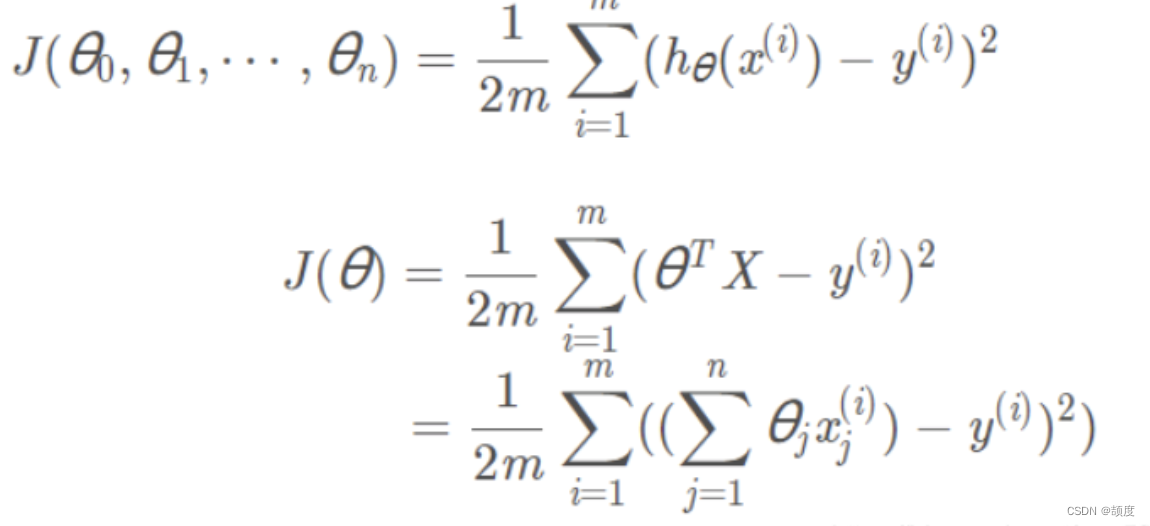运用梯度下降：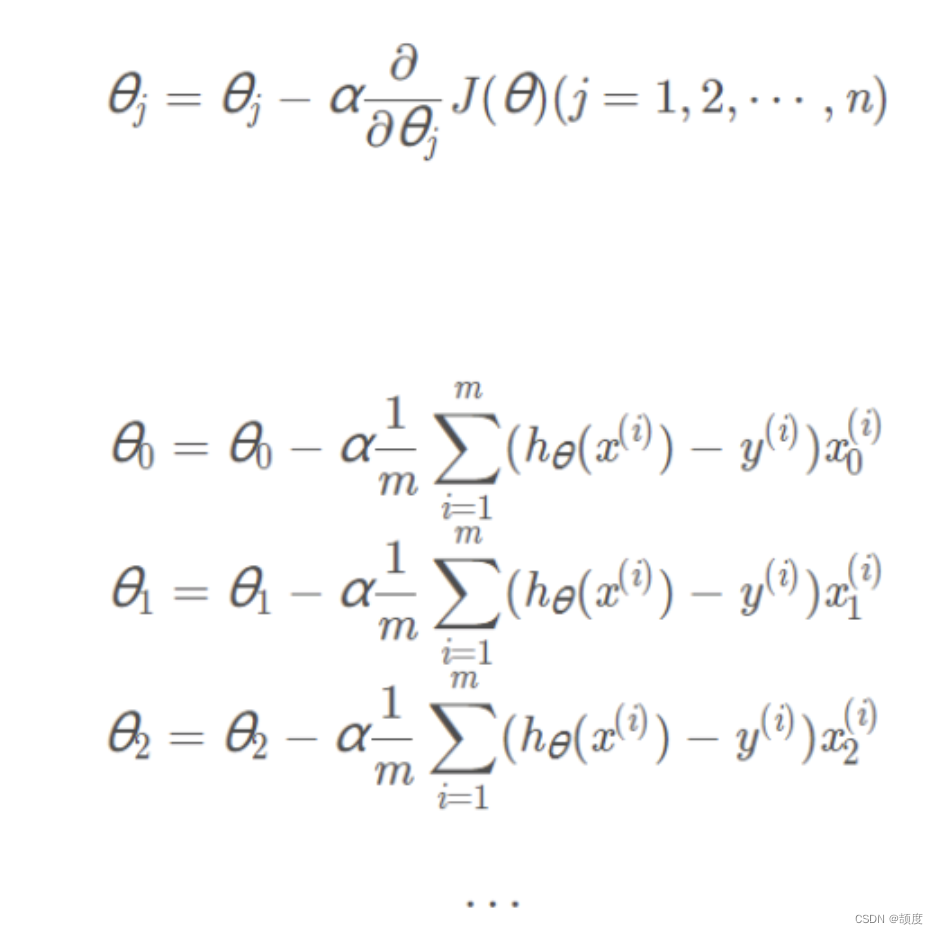四、鸢尾花数据集

Iris 鸢尾花数据集内包含 3 类，分别为山鸢尾（Iris-setosa）、变色鸢尾（Iris-versicolor）和维吉尼亚鸢尾（Iris-virginica），共 150 条记录，每类各 50 个数据，每条记录都有 4 项特征：花萼长度、花萼宽度、花瓣长度、花瓣宽度，可以通过这 4 个特征预测鸢尾花卉属于哪一品种。 这是本文章所使用的鸢尾花数据集： sl：花萼长度 sw：花萼宽度 pl：花瓣长度 pw：花瓣宽度； type：类别：（Iris-setosaIris-versicolorIris-virginica 三类）

部分展示：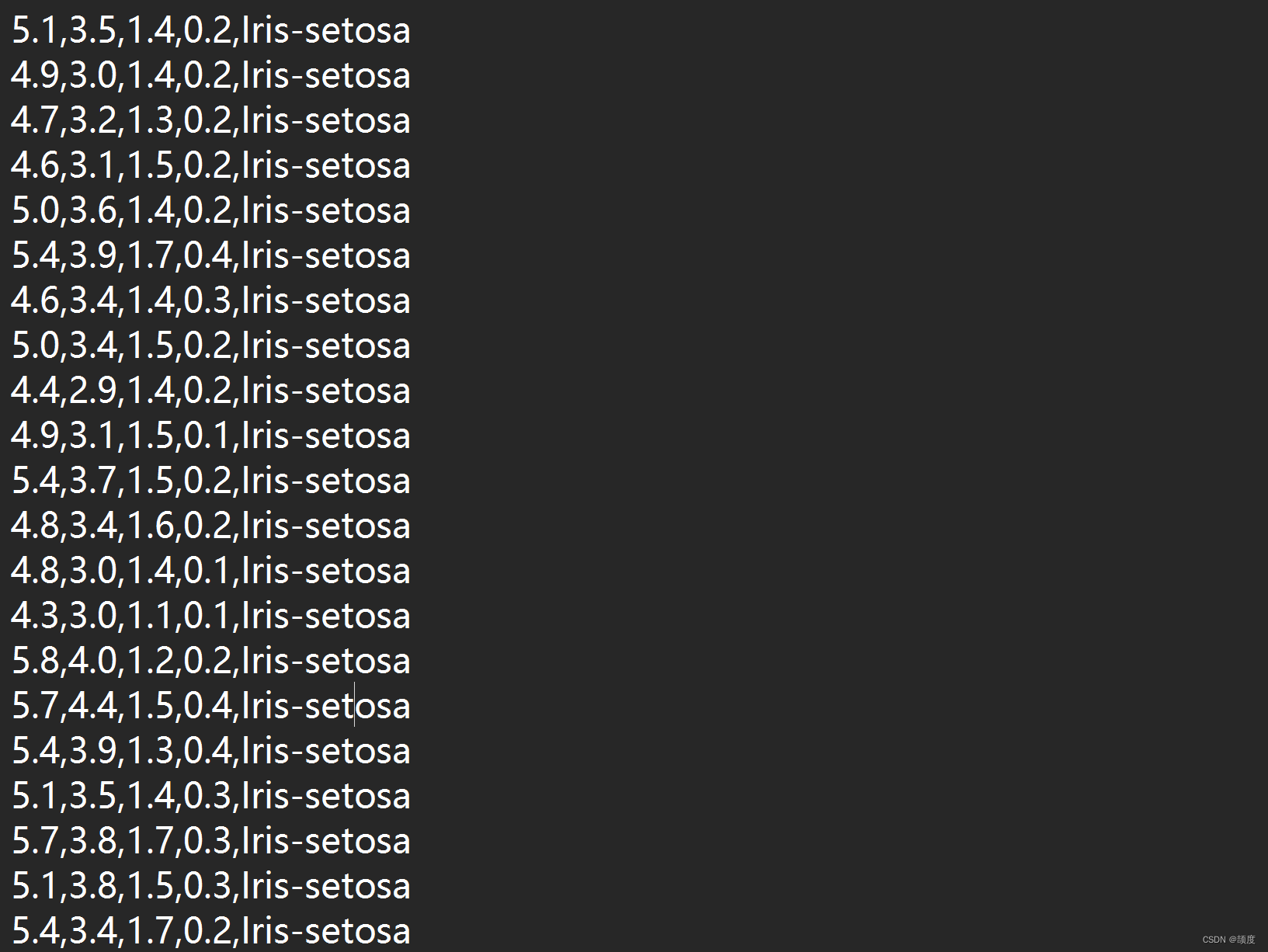代码：

import pandas as pd
import numpy as np
import time
import random
def MGD_train(X, y, alpha=0.0001, maxIter=1000, theta_old=None):
'''
MGD训练线性回归
传入:
X       :  已知数据
y       :  标签
alpha   :  学习率
maxIter :  总迭代次数
返回:
theta : 权重参数
'''
# 初始化权重参数
theta = np.ones(shape=(X.shape,))
if not theta_old is None:
# 假装是断点续训练
theta = theta_old.copy()

# axis=1 表示横轴，方向从左到右；axis=0 表示纵轴，方向从上到下
for i in range(maxIter):
# 预测
y_pred = np.sum(X * theta, axis=1)
# 全部数据得到的梯度
gradient = np.average((y - y_pred).reshape(-1, 1) * X, axis=0)
# 更新学习率
return theta

def SGD_train(X, y, alpha=0.0001, maxIter=1000, theta_old=None):
'''
SGD训练线性回归
传入:
X       :  已知数据
y       :  标签
alpha   :  学习率
maxIter :  总迭代次数

返回:
theta : 权重参数
'''
# 初始化权重参数
theta = np.ones(shape=(X.shape,))
if not theta_old is None:
# 假装是断点续训练
theta = theta_old.copy()
# 数据数量
data_length = X.shape
for i in range(maxIter):
# 随机选择一个数据
index = np.random.randint(0, data_length)
# 预测
y_pred = np.sum(X[index, :] * theta)
# 一条数据得到的梯度
gradient = (y[index] - y_pred) * X[index, :]
# 更新学习率
return theta

def MBGD_train(X, y, alpha=0.0001, maxIter=1000, batch_size=10, theta_old=None):
'''
MBGD训练线性回归
传入:
X          :  已知数据
y          :  标签
alpha      :  学习率
maxIter    :  总迭代次数
batch_size :  没一轮喂入的数据数

返回:
theta : 权重参数
'''
# 初始化权重参数
theta = np.ones(shape=(X.shape,))

if not theta_old is None:
# 假装是断点续训练
theta = theta_old.copy()

# 所有数据的集合
all_data = np.concatenate([X, y.reshape(-1, 1)], axis=1)
for i in range(maxIter):
# 从全部数据里选 batch_size 个 item
X_batch_size = np.array(random.choices(all_data, k=batch_size))

# 重新给 X, y 赋值
X_new = X_batch_size[:, :-1]
y_new = X_batch_size[:, -1]

# 将数据喂入，更新 theta
theta = MGD_train(X_new, y_new, alpha=0.0001, maxIter=1, theta_old=theta)
return theta

def GD_predict(X, theta):
'''
用于预测的函数
传入:
X     : 数据
theta : 权重
返回:
y_pred: 预测向量
'''
y_pred = np.sum(theta * X, axis=1)
# 实数域空间 -> 离散三值空间，则需要四舍五入
y_pred = (y_pred + 0.5).astype(int)
return y_pred

def calc_accuracy(y, y_pred):
'''
计算准确率
传入:
y        : 标签
y_pred   : 预测值
返回:
accuracy : 准确率
'''
return np.average(y == y_pred) * 100
# 读取数据
iris_raw_data = pd.read_csv('iris.data', names  =['sepal length', 'sepal width', 'petal length', 'petal width', 'class'])
# 将三种类型映射成整数
Iris_dir = {'Iris-setosa': 1, 'Iris-versicolor': 2, 'Iris-virginica': 3}
iris_raw_data['class'] = iris_raw_data['class'].apply(lambda x:Iris_dir[x])
# 训练数据 X
iris_data = iris_raw_data.values[:, :-1]
# 标签 y
y = iris_raw_data.values[:, -1]
# 用 MGD 训练的参数
start = time.time()
theta_MGD = MGD_train(iris_data, y)
run_time = time.time() - start
y_pred_MGD = GD_predict(iris_data, theta_MGD)
print("MGD训练1000轮得到的准确率{:.2f}% 运行时间是{:.2f}s".format(calc_accuracy(y, y_pred_MGD), run_time))
# 用 SGD 训练的参数
start = time.time()
theta_SGD = SGD_train(iris_data, y)
run_time = time.time() - start
y_pred_SGD = GD_predict(iris_data, theta_SGD)
print("SGD训练1000轮得到的准确率{:.2f}% 运行时间是{:.2f}s".format(calc_accuracy(y, y_pred_SGD), run_time))
# 用 MBGD 训练的参数
start = time.time()
theta_MBGD = MBGD_train(iris_data, y)
run_time = time.time() - start
y_pred_MBGD = GD_predict(iris_data, theta_MBGD)
print("MBGD训练1000轮得到的准确率{:.2f}% 运行时间是{:.2f}s".format(calc_accuracy(y, y_pred_MBGD), run_time))

结果：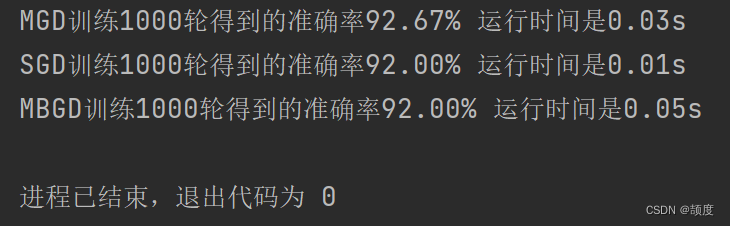展开全文机器学习 python 人工智能 算法
• 文章目录一、多元线性回归模型预测房价1.基础包导入2.变量探索3.多元线性回归建模4.模型优化二、用Excel重做上面的多元线性回归，求解回归方程三、用机器学习库Sklearn库重做上面的多元线性归四、参考文献 一、多元...

# 1.基础包导入

import pandas as pd
import numpy as np
import seaborn as sns
import matplotlib.pyplot as plt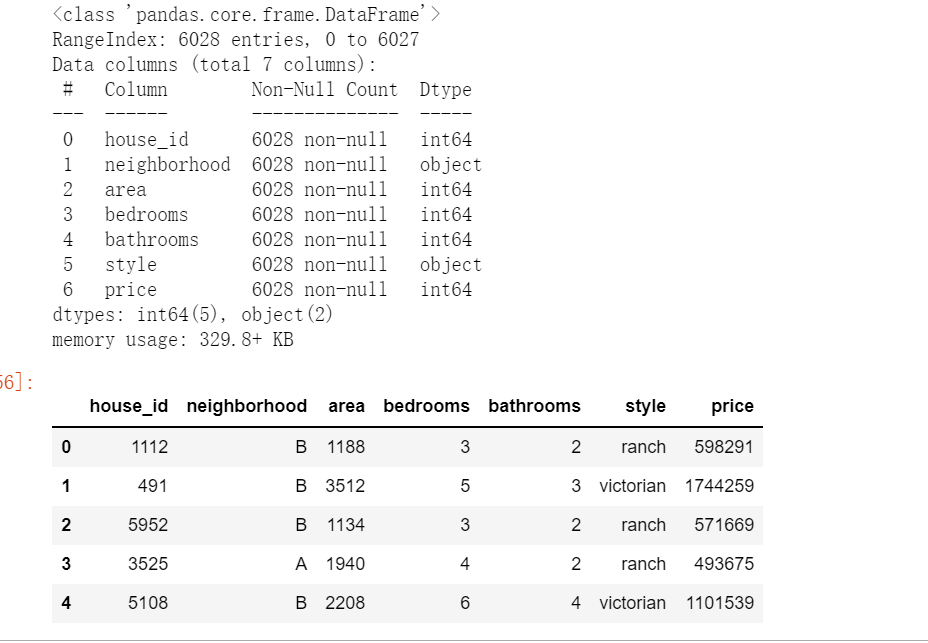# 2.变量探索

# 异常值处理
# ================ 异常值检验函数：iqr & z分数 两种方法 =========================
def outlier_test(data, column, method=None, z=2):
""" 以某列为依据，使用 上下截断点法 检测异常值(索引) """
"""
full_data: 完整数据
column: full_data 中的指定行，格式 'x' 带引号
return 可选; outlier: 异常值数据框
upper: 上截断点; lower: 下截断点
method：检验异常值的方法（可选, 默认的 None 为上下截断点法），
选 Z 方法时，Z 默认为 2
"""
# ================== 上下截断点法检验异常值 ==============================
if method == None:
print(f'以 {column} 列为依据，使用 上下截断点法(iqr) 检测异常值...')
print('=' * 70)
# 四分位点；这里调用函数会存在异常
column_iqr = np.quantile(data[column], 0.75) - np.quantile(data[column], 0.25)
# 1，3 分位数
(q1, q3) = np.quantile(data[column], 0.25), np.quantile(data[column], 0.75)
# 计算上下截断点
upper, lower = (q3 + 1.5 * column_iqr), (q1 - 1.5 * column_iqr)
# 检测异常值
outlier = data[(data[column] <= lower) | (data[column] >= upper)]
print(f'第一分位数: {q1}, 第三分位数：{q3}, 四分位极差：{column_iqr}')
print(f"上截断点：{upper}, 下截断点：{lower}")
return outlier, upper, lower
# ===================== Z 分数检验异常值 ==========================
if method == 'z':
""" 以某列为依据，传入数据与希望分段的 z 分数点，返回异常值索引与所在数据框 """
"""
params
data: 完整数据
column: 指定的检测列
z: Z分位数, 默认为2，根据 z分数-正态曲线表，可知取左右两端的 2%，
根据您 z 分数的正负设置。也可以任意更改，知道任意顶端百分比的数据集合
"""
print(f'以 {column} 列为依据，使用 Z 分数法，z 分位数取 {z} 来检测异常值...')
print('=' * 70)
# 计算两个 Z 分数的数值点
mean, std = np.mean(data[column]), np.std(data[column])
upper, lower = (mean + z * std), (mean - z * std)
print(f"取 {z} 个 Z分数：大于 {upper} 或小于 {lower} 的即可被视为异常值。")
print('=' * 70)
# 检测异常值
outlier = data[(data[column] <= lower) | (data[column] >= upper)]
return outlier, upper, lower

outlier, upper, lower = outlier_test(data=df, column='price', method='z')
outlier.info(); outlier.sample(5)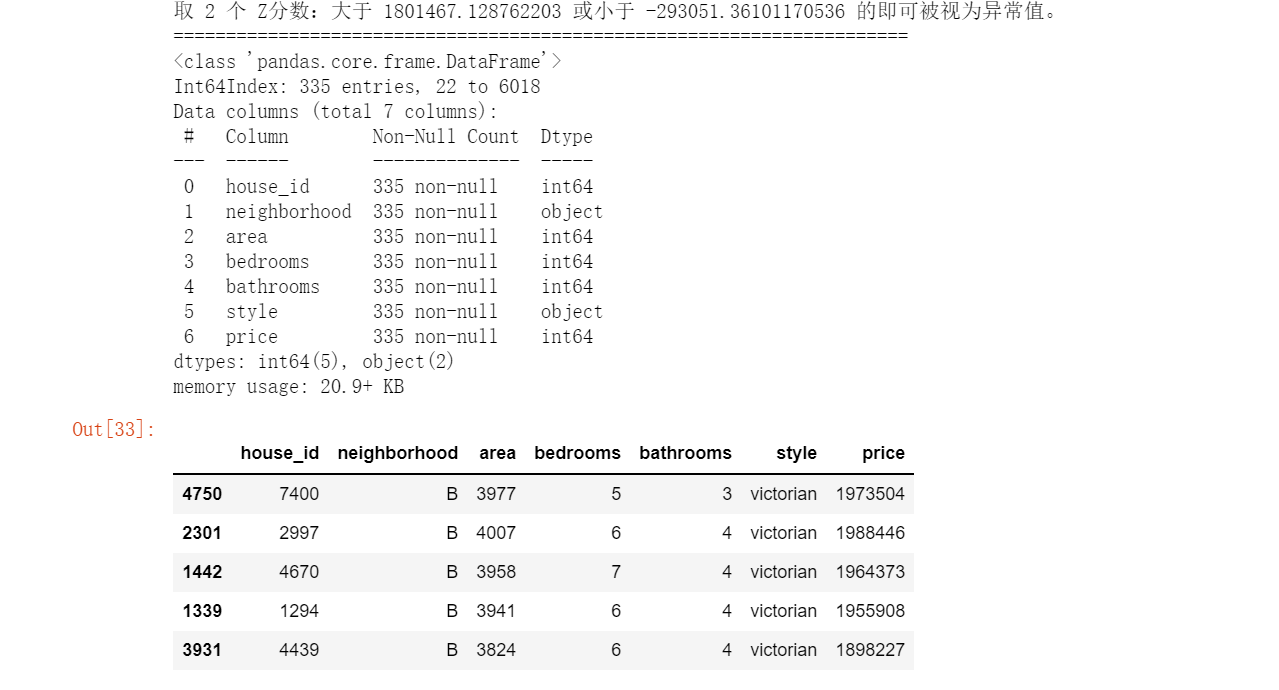# 这里简单的丢弃即可
df.drop(index=outlier.index, inplace=True)

# 类别变量，又称为名义变量，nominal variables
nominal_vars = ['neighborhood', 'style']
for each in nominal_vars:
print(each, ':')
print(df[each].agg(['value_counts']).T)
# 直接 .value_counts().T 无法实现下面的效果
## 必须得 agg，而且里面的中括号 [] 也不能少
print('='*35)
# 发现各类别的数量也都还可以，为下面的方差分析做准备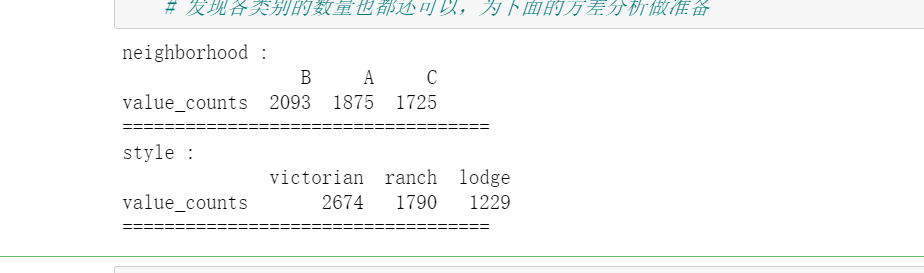# 热力图
def heatmap(data, method='pearson', camp='RdYlGn', figsize=(10 ,8)):
"""
data: 整份数据
method：默认为 pearson 系数
camp：默认为：RdYlGn-红黄蓝；YlGnBu-黄绿蓝；Blues/Greens 也是不错的选择
figsize: 默认为 10，8
"""
## 消除斜对角颜色重复的色块
plt.figure(figsize=figsize, dpi= 80)
sns.heatmap(data.corr(method=method), \
xticklabels=data.corr(method=method).columns, \
yticklabels=data.corr(method=method).columns, cmap=camp, \
center=0, annot=True)

# 通过热力图可以看出 area，bedrooms，bathrooms 等变量与房屋价格 price 的关系都还比较强
## 所以值得放入模型，但分类变量 style 与 neighborhood 两者与 price 的关系未知
heatmap(data=df, figsize=(6,5))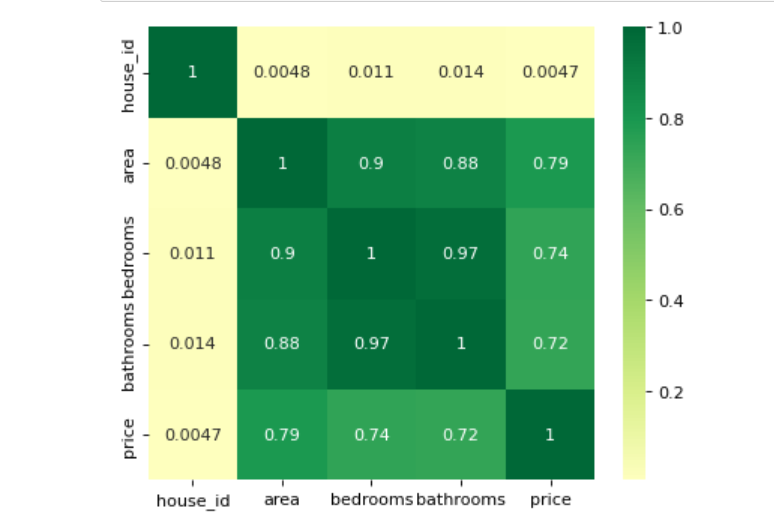# 刚才的探索我们发现，style 与 neighborhood 的类别都是三类，
## 如果只是两类的话我们可以进行卡方检验，所以这里我们使用方差分析

## 利用回归模型中的方差分析
## 只有 statsmodels 有方差分析库
## 从线性回归结果中提取方差分析结果
import statsmodels.api as sm
from statsmodels.formula.api import ols # ols 为建立线性回归模型的统计学库
from statsmodels.stats.anova import anova_lm


插播一条样本量和置信水平 α_level 的注意点（置信水平 α 的选择经验）

样本量 α-level
≤ 100 10%
100 ＜ n ≤ 500 5%
500 ＜ n ≤ 1000 1%
n ＞ 2000 千分之一


样本量过大，α-level 就没什么意义了。
数据量很大时，p 值就没用了，样本量通常不超过 5000，
为了证明两变量间的关系是稳定的，样本量要控制好。

# 数据集样本数量：6028，这里随机选择 600 条，如果希望分层抽样，可参考文章：
df = df.copy().sample(600)
# C 表示告诉 Python 这是分类变量，否则 Python 会当成连续变量使用
## 这里直接使用方差分析对所有分类变量进行检验
## 下面几行代码便是使用统计学库进行方差分析的标准姿势
lm = ols('price ~ C(neighborhood) + C(style)', data=df).fit()
anova_lm(lm)
# Residual 行表示模型不能解释的组内的，其他的是能解释的组间的
# df: 自由度（n-1）- 分类变量中的类别个数减1
# sum_sq: 总平方和（SSM），residual行的 sum_eq: SSE
# mean_sq: msm, residual行的 mean_sq: mse
# F：F 统计量，查看卡方分布表即可
# PR(>F): P 值
# 反复刷新几次，发现都很显著，所以这两个变量也挺值得放入模型中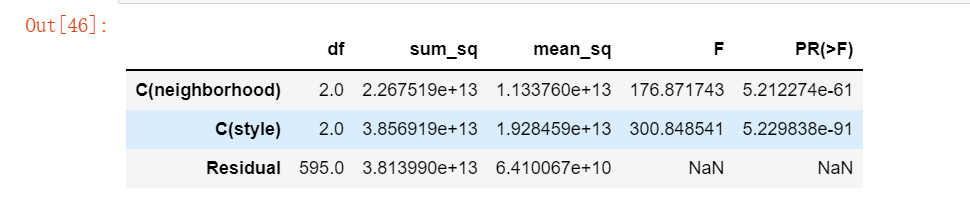# 3.多元线性回归建模

from statsmodels.formula.api import ols
lm = ols('price ~ area + bedrooms + bathrooms', data=df).fit()
lm.summary()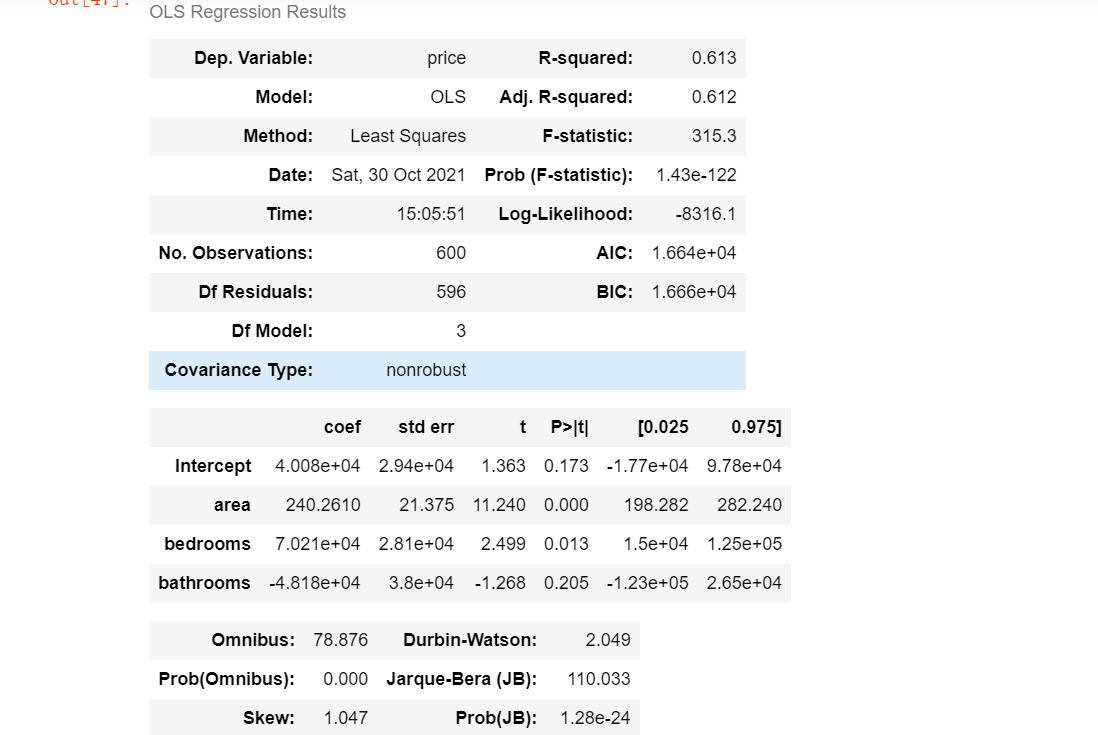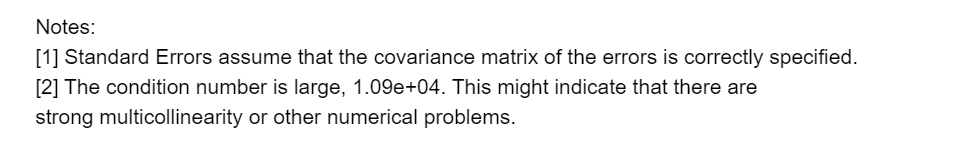# 4.模型优化

发现精度还不够高，这里通过添加虚拟变量与使用方差膨胀因子检测多元共线性的方式来提升模型精度

# 设置虚拟变量
# 以名义变量 neighborhood 街区为例
nominal_data = df['neighborhood']
# 设置虚拟变量
dummies = pd.get_dummies(nominal_data)
dummies.sample() # pandas 会自动帮你命名
# 每个名义变量生成的虚拟变量中，需要各丢弃一个，这里以丢弃C为例
dummies.drop(columns=['C'], inplace=True)
dummies.sample()# 将结果与原数据集拼接
results = pd.concat(objs=[df, dummies], axis='columns') # 按照列来合并
results.sample(3)
# 对名义变量 style 的处理可自行尝试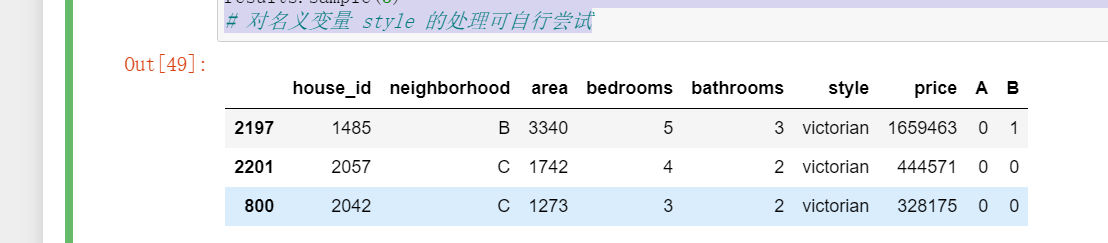# 再次建模
lm = ols('price ~ area + bedrooms + bathrooms + A + B', data=results).fit()
lm.summary()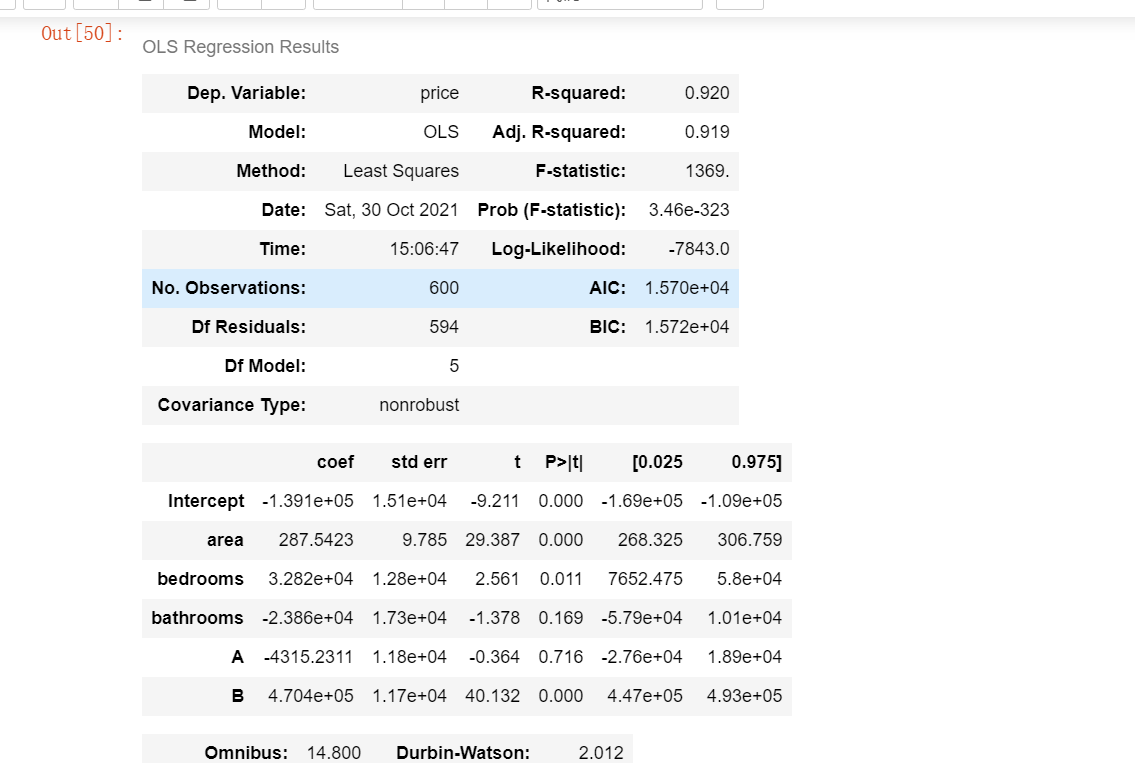# 自定义方差膨胀因子的检测公式
def vif(df, col_i):
"""
df: 整份数据
col_i：被检测的列名
"""
cols = list(df.columns)
cols.remove(col_i)
cols_noti = cols
formula = col_i + '~' + '+'.join(cols_noti)
r2 = ols(formula, df).fit().rsquared
return 1. / (1. - r2)

test_data = results[['area', 'bedrooms', 'bathrooms', 'A', 'B']]
for i in test_data.columns:
print(i, '\t', vif(df=test_data, col_i=i))
# 发现 bedrooms 和 bathrooms 存在强相关性，可能这两个变量是解释同一个问题# 再次进行多元共线性检测
test_data = df[['area', 'bathrooms']]
for i in test_data.columns:
print(i, '\t', vif(df=test_data, col_i=i))# 二、用Excel重做上面的多元线性回归，求解回归方程

基于多元线性回归的房价预测
摘要

市场房价的走向受到多种因素的影响，通过对影响市场房价的多种因素进行分析，有助于对未来房价的走势进行较为准确的评估。
多元线性回归适用于对受到多因素影响的数据进行分析的场景。由多个自变量的最优组合共同来预测或估计因变量，比只用一个自变量进行预测或估计更有效，更符合实际。本文基于数学模型，对过去一段时间某一地区的房屋出售价格等相关数据进行整理，利用多元线性回归的方法对数据进行分析，预测该地区未来的房价走势。
关键词：多元线性回归；房价预测；数据分析；

引言
对未来房价进行预测，在一定程度上影响着社会经济的发展。广义上讲，精确的房价预测有助于国家对市场房价走势的宏观调控，小范围来讲，未来房价预测是企业战略规划的一部分，对于消费者而言，房价预测为个人经济的合理规划起到了积极作用。由于房屋售价与多因素有关，并且房屋价格与影响房价的一些因素存在线性关系，所以选取多元线性回归模型研究该问题较为合适。
本次课题研究通过对某段时间某地区的已售房价数据进行线性回归分析，探索影响房价高低的主要因素，并对这些影响因素的影响程度进行分析，利用分析得到的数据，对未来房价的趋势和走向进行预测。

线性回归理论基础
一元线性回归是分析只有一个自变量（自变量x和因变量y）线性相关关系的方法。一元线性回归分析的数学模型为：y = a+bx+ε。
使用偏差平方和分别对参数a和参数b求偏导，可以得到线性模型的未知参数a、b的最小二乘估计值，其中，偏差平方和定义为∑(yi-a-bXi)2，a和b的唯一解如图所示。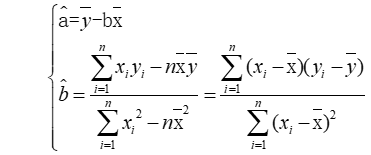** 参数的最小二乘估计**
为了方便回归效果显著性检验，根据b的估计，引入LXX、LYY、LXY三个数学符号，这三个数学符号定义如图所示。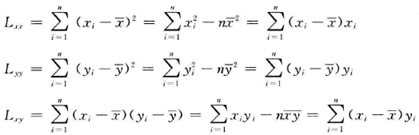图 LXX、LYY、LXY的数学定义
在现实问题研究中，因变量的变化往往受几个重要因素的影响，此时就需要用两个或两个以上的影响因素作为自变量来解释因变量的变化，这就是多元回归。也就是说，当多个自变量与因变量之间是线性关系时，所进行的回归分析就是多元性回归。多元线性回归的数学模型为：y=β0+β1X1+β2X2+…++βpXp+ε。使用残差平方和分别对参数βi（i=0,1,…,p）求偏导，可以得到线性模型的未知参数βi（i=0,1,…,p）的估计值，β矩阵的估计值如图所示。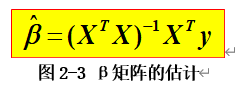回归效果的显著性检验
对平面上杂乱无章的点，利用最小二乘法求解出的线性回归方程是毫无意义的，线性回归反映出的趋势描述是否合理，需要一个数量指标来度量。
数据总的波动可以用离差平方和LYY来描述。它表示y的各次离差yi-y ̅的平方和。LYY数值越大，说明yi数值波动越大，也就是越分散。离差平方和LYY可以分解为回归直线上y的离差平方和U以及yi与回归直线上的y间的差的平方和Q。其中，U是由于x对y的线性相关关系引起的y的分散性，Q是由随机误差引起的分散性。yi-y ̅分解如图2-4所示。在总和中，U所占比重越大，说明随机误差所占的比重越小，回归效果越显著。故此，可以使用决定系数R2来度量线性回归效果是否显著，R2作为拟合优度，表示用直线来拟合数据的好坏，R2等于U/Lyy。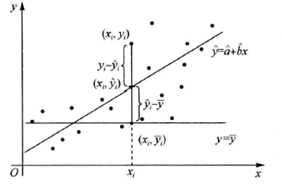R2开方后的结果为皮尔逊相关系数，皮尔逊（Pearson）相关系数可以用来衡量两个数据集合是否在一条线上面，从而衡量定距变量间的线性关系。相关系数的绝对值越大，相关性越强；相关系数越接近于1或-1，相关度越强，相关系数越接近于0，相关度越弱。当|r|>=0.8时，x和y强相关，当|r|<0.3时，x和y弱相关。皮尔逊相关系数定义为如图所示。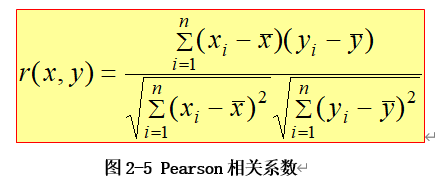对于一元线性回归模型，线性回归模型效果的显著性可以通过假设检验问题H0：b=0；H1：b≠0进行判断，检验方法包括F检验法和t检验法。F检验属于回归方程显著性检验，是检验x与y是否相关的检验方法。t检验是回归系数显著性检验，是检验变量x是否有用的方法。H0成立时，两种检验方法定义如图2-6、图2-7所示。H0不成立时，对于给定的显著性水平α，当F>F1-α(1,n-2)时，回归效果显著。当|t|>t1-α/2(n-2)时，认为回归系数影响显著，否则回归系数的效果不显著。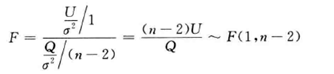一元线性回归F检验法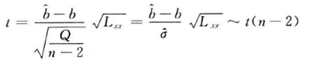一元线性回归t检验法
对于多元线性回归模型，回归效果的显著性可以使用F检验法通过假设检验问题H0：β0=β1=β2=…=βp=0；H1：βi（i=0,1,…,p）不全为0进行判断，H0成立时，F检验方法定义如图2-8所示。H0不成立时，对于给定的显著性水平α，当F>F1-α(p,n-p-1)时，回归效果显著。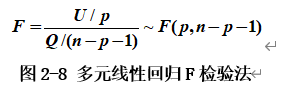回归系数的显著性检验可以使用t检验法通过假设检验问题H0：βi=0；H1：βi≠0进行判断。H0成立时，t检验方法定义如图2-9所示。H0不成立时，对于给定的显著性水平α，当|t|>t1-α/2(n-p-1)时，认为回归系数影响显著，否则回归系数的效果不显著。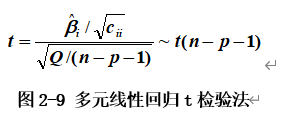基于机器学习的线性回归与梯度下降
机器学习横跨计算机科学、工程技术和统计学等多个学科，渗透到了人们生产和生活中的各个领域，被广泛应用于各行各业之中，在当今世界激烈的竞争中，掌握和理解机器学习的基础模型和基本方法是非常有必要的。
机器学习中的线性回归模型以数理统计的线性回归模型为基础，它用一条直线对数据点进行拟合，在机器学习中，回归问题的求解过程就是寻找最佳拟合参数集的过程，也就是寻找满足使得估计值与实际值的方差最小时的参数解，这个过程用到了损失函数，损失函数定义如图2-10所示。利用损失函数，可以求解最佳拟合参数集。利用损失函数进行求解可以采用梯度下降法。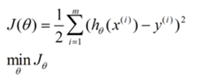梯度下降法的计算过程就是沿梯度下降的方向求解极小值或沿梯度上升方向求解极大值。一般情况下，梯度向量为0的话说明是到了一个极值点，此时梯度的幅值也为0。采用梯度下降算法进行最优化求解时，算法迭代的终止条件是梯度向量的幅值接近0或接近一个非常小的常数阈值。梯度下降的过程如图所示。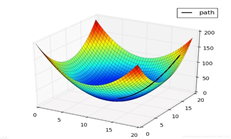使用EXCEL进行数据分析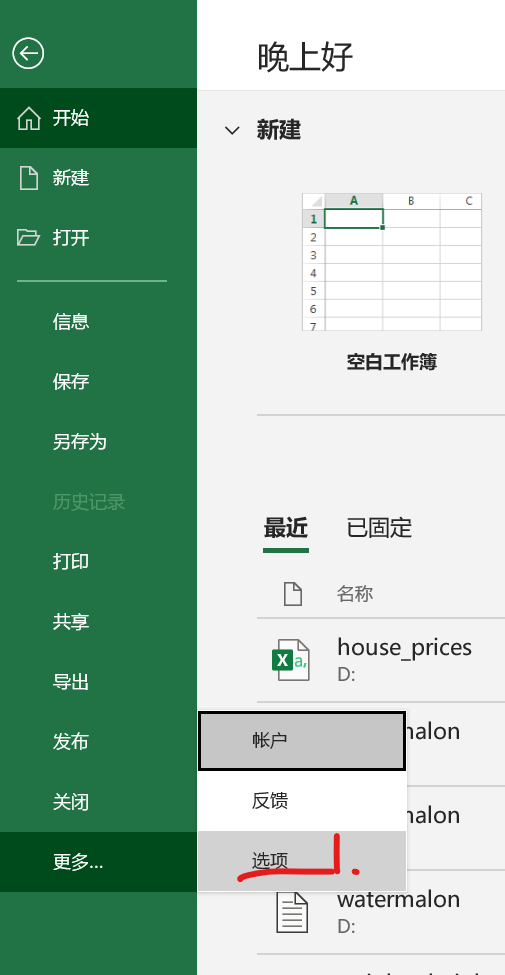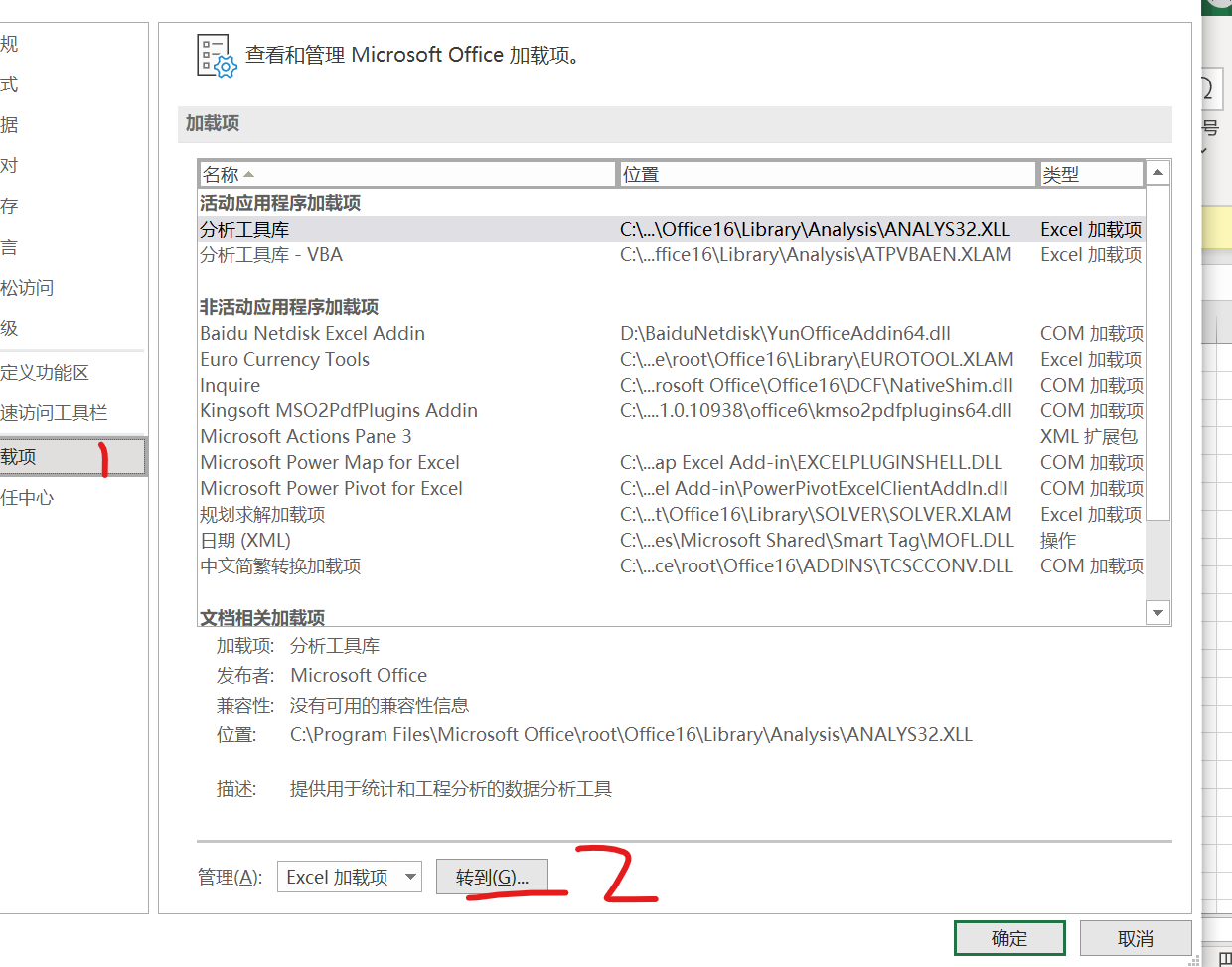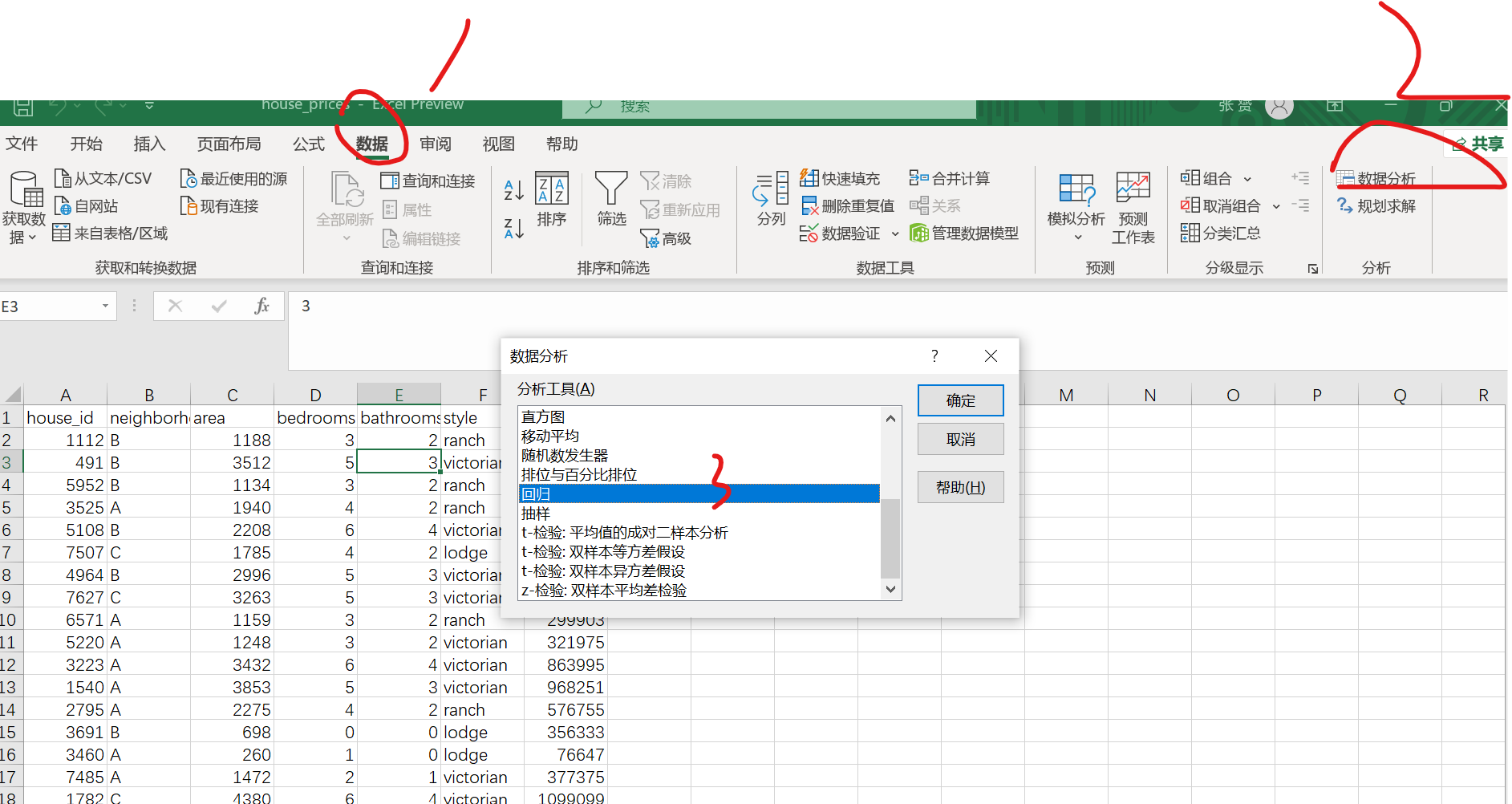选择x,y值域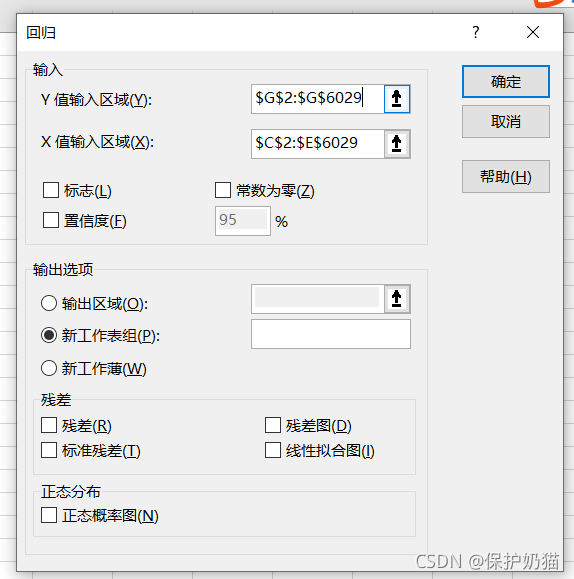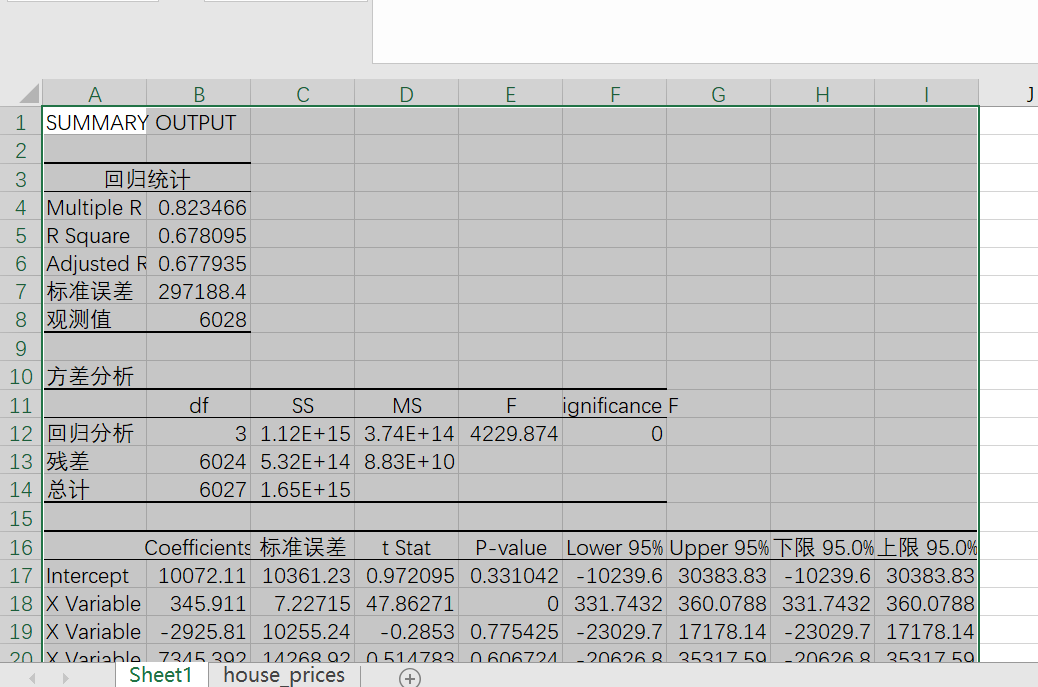字段Multiple R代表复相关系数R，也就是R2的平方根，又称相关系数，用来衡量自变量x与y之间的相关程度的大小。
R Square是复测定系数，也就是相关系数R的平方。
标准误差用来衡量拟合程度的大小，也用于计算与回归相关的其它统计量，此值越小，说明拟合程度越好。
观察值是用于估计回归方程的数据的观察值个数，本次数据集共有20条数据，所以观察值为20。
Coefficients为常数项

设因变量房屋售价price为y，自变量面积area为x1，bedrooms为x2，bathrooms为x3

所以得到方程：
y=10072.11+345.911x1-2925.81x2+7345.392x3

# 三、用机器学习库Sklearn库重做上面的多元线性归

1. 不处理直接求解

import pandas as pd
import numpy as np
import math
import matplotlib.pyplot as plt #画图
from sklearn import linear_model #线性模型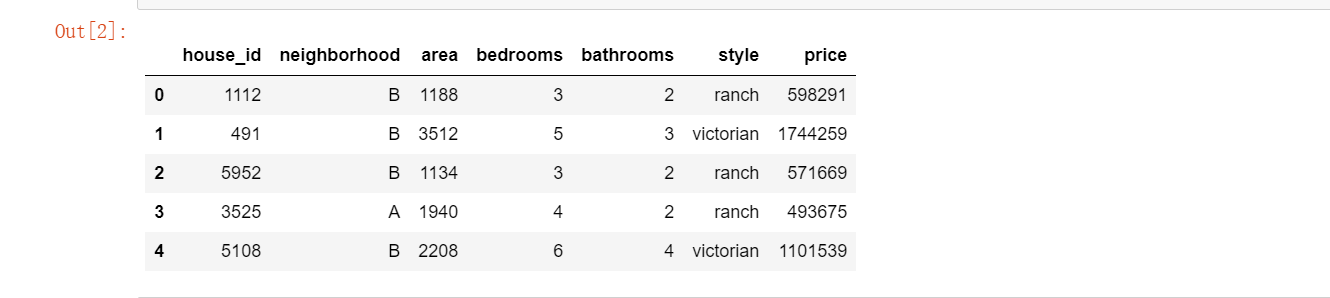new_data=data.iloc[:,1:]#除掉house_id这一列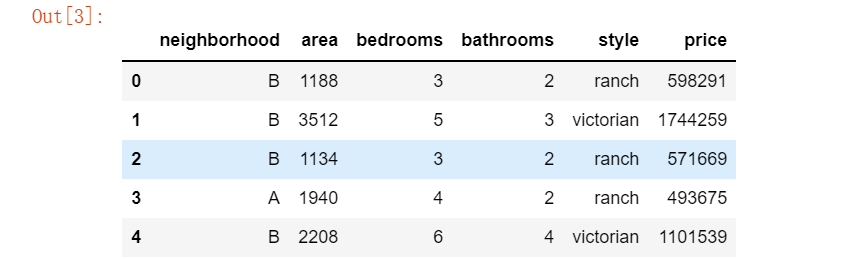new_data.corr()#相关系数矩阵，只统计数值列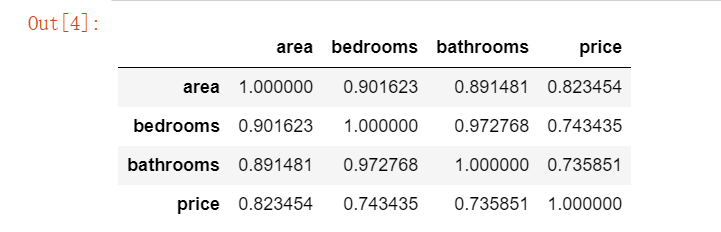"""取are、bedrooms和bathroom作为X,price为Y求线性回归。"""
x_data = new_data.iloc[:, 1:4] #are、bedrooms、bathroom对应列
y_data = new_data.iloc[:, -1] #price对应列
print(x_data, y_data, len(x_data))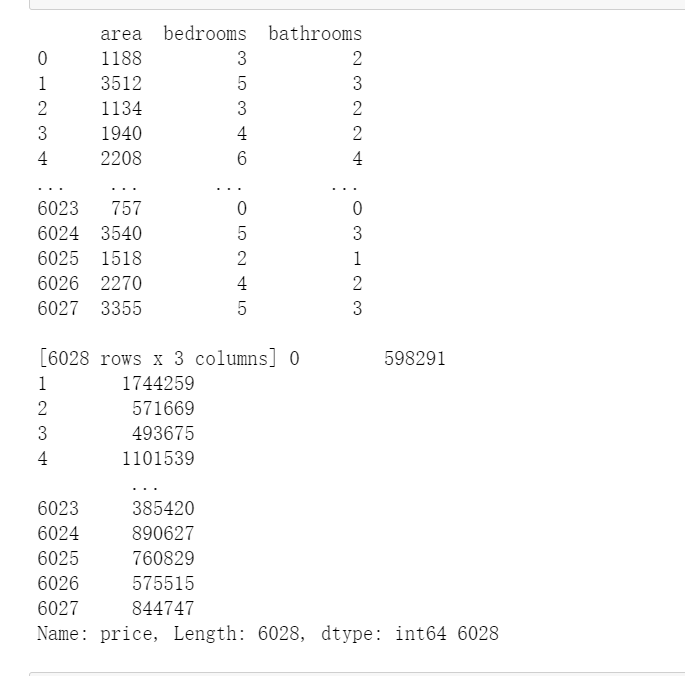# 应用模型
model = linear_model.LinearRegression()
model.fit(x_data, y_data)
print("回归系数：", model.coef_)
print("截距：", model.intercept_)
print('回归方程: price=',model.coef_,'*area +',model.coef_,'*bedrooms +',model.coef_,'*bathromms +',model.intercept_)2 数据进行清洗后再求解

new_data_Z=new_data.iloc[:,0:]
new_data_IQR=new_data.iloc[:,0:]
def outlier_test(data, column, method=None, z=2):

if method == None:
print(f'以 {column} 列为依据，使用 上下截断点法(iqr) 检测异常值...')
print('=' * 70)
column_iqr = np.quantile(data[column], 0.75) - np.quantile(data[column], 0.25)
(q1, q3) = np.quantile(data[column], 0.25), np.quantile(data[column], 0.75)
upper, lower = (q3 + 1.5 * column_iqr), (q1 - 1.5 * column_iqr)
outlier = data[(data[column] <= lower) | (data[column] >= upper)]
print(f'第一分位数: {q1}, 第三分位数：{q3}, 四分位极差：{column_iqr}')
print(f"上截断点：{upper}, 下截断点：{lower}")
return outlier, upper, lower

if method == 'z':

print(f'以 {column} 列为依据，使用 Z 分数法，z 分位数取 {z} 来检测异常值...')
print('=' * 70)
mean, std = np.mean(data[column]), np.std(data[column])
upper, lower = (mean + z * std), (mean - z * std)
print(f"取 {z} 个 Z分数：大于 {upper} 或小于 {lower} 的即可被视为异常值。")
print('=' * 70)
outlier = data[(data[column] <= lower) | (data[column] >= upper)]
return outlier, upper, lower

outlier, upper, lower = outlier_test(data=new_data_Z, column='price', method='z')
outlier.info(); outlier.sample(5)

#这里简单丢弃即可
new_data_Z.drop(index=outlier.index, inplace=True)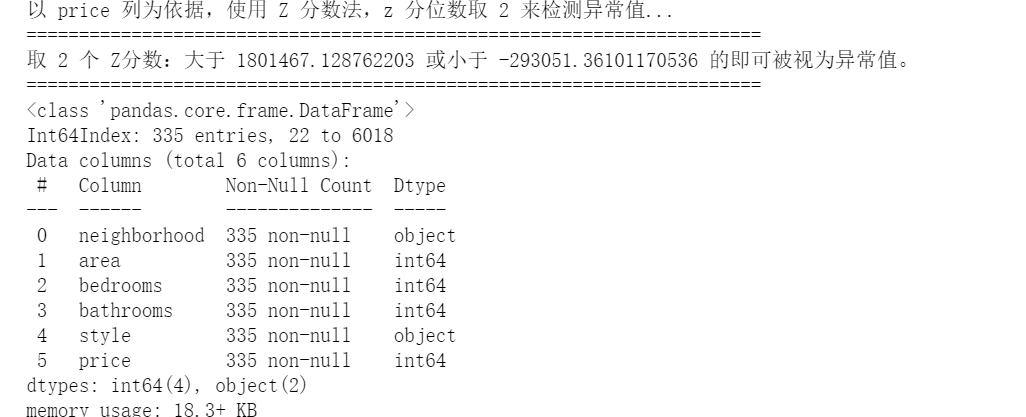outlier, upper, lower = outlier_test(data=new_data_IQR, column='price')
outlier.info(); outlier.sample(5)

# 这里简单的丢弃即可
new_data_IQR.drop(index=outlier.index, inplace=True)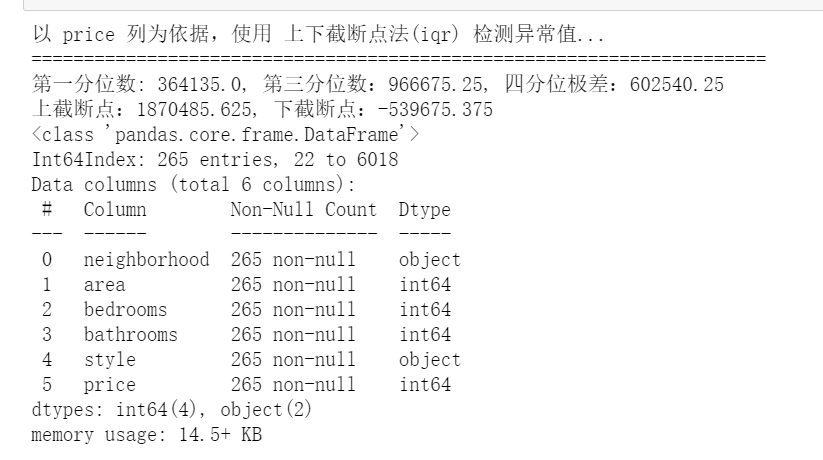print("原数据相关性矩阵")
new_data.corr()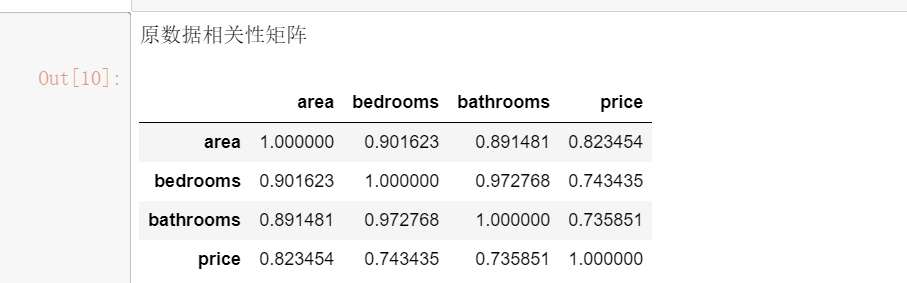print("z方法处理的相关性矩阵")
new_data_Z.corr()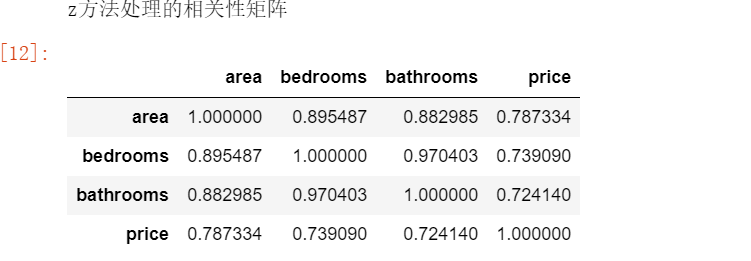print("IQR方法处理的数据相关性矩阵")
new_data_IQR.corr()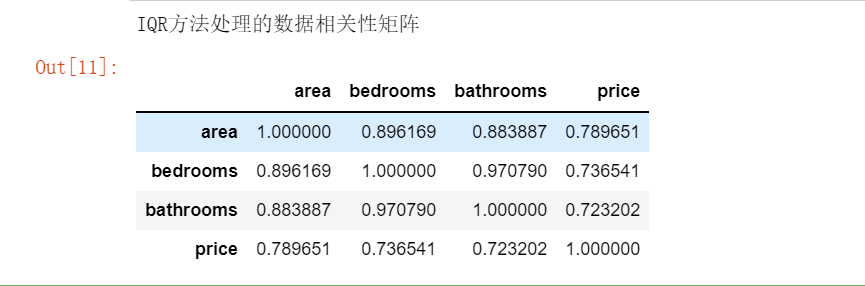x_data = new_data_Z.iloc[:, 1:4]
y_data = new_data_Z.iloc[:, -1]
# 应用模型
model = linear_model.LinearRegression()
model.fit(x_data, y_data)
print("回归系数：", model.coef_)
print("截距：", model.intercept_)
print('回归方程: price=',model.coef_,'*area +',model.coef_,'*bedrooms +',model.coef_,'*bathromms +',model.intercept_)x_data = new_data_IQR.iloc[:, 1:4]
y_data = new_data_IQR.iloc[:, -1]
# 应用模型
model = linear_model.LinearRegression()
model.fit(x_data, y_data)
print("回归系数：", model.coef_)
print("截距：", model.intercept_)
print('回归方程: price=',model.coef_,'*area +',model.coef_,'*bedrooms +',model.coef_,'*bathromms +',model.intercept_)3.3 对比

不做任何数据处理： price= 345.911018840024 *area +
-2925.806324666705 *bedrooms + 7345.391713693825 *bathromms    + 10072.107046726742

Z方式清理数据：price= 226.4211697383351 *area + 49931.50311720713 *bedrooms + -12224.71724496588 *bathromms + 64356.04135007458

IQR方式清理数据：price= 242.6111551782956 *area + 41547.43068790577 *bedrooms + -6415.78250090158 *bathromms + 58018.13845504692


# 四、参考文献

https://blog.csdn.net/qq_55691662/article/details/120960932

https://blog.csdn.net/weixin_43196118/article/details/108462140

https://blog.csdn.net/m0_51120713/article/details/120969812

https://blog.csdn.net/weixin_43196118/article/details/108462140

展开全文python 算法
• 多元线性回归预测代码，读取EXCLE表格，设置训练数据集合测试数据集，评价方式采用相关系数R2和均方根误差RMSE
• 多元线性回归模型是一种简单而且有效的数学模型，一直在各领域广泛使用。一个多元回归模型建好后，如何评价模型的优劣呢？1. F值检验因变量的总变异(数据与均值之差的平方和，记为SStotal)由回归平方和(因变量的变异...
• 用matlab对多元还原概率预测代码线性回归模型解释 这是一个包含使用Sklearn、pandas、Numpy 和 Seaborn进行线性回归的解释的存储库。 还执行探索性数据分析（EDA）和可视化。 本说明分为以下部分，我们将详细介绍每...
• #多元线性回归预测房子的价格，构建一个房子价格的python模型。 ##ex1data2.txt中包含了房子价格的训练组。第一列是房子的尺寸（平方英尺），第二列是卧室的数量，第三列是房子的价格。
• 利用线性回归预测工资与经验的关系，多元线性回归预测产品利润与各个因素，如投入，地点的关系
• 系统聚类和多元线性回归模型的物流需求分析，孙淑生，黄宝军，物流业作为经济发展的基石，是衡量一个区域综合经济实力和科技水平的重要标志。为了更好地能够在未来某一时段内的准确预测将成为
• 一、公式推导，矩阵最小二乘法(参考高等代数) 这部分知识可以参考： 高等代数9 7 向量到子空间的距离 最小二乘法 - ...如果是实现非线性 这部分知识可以参考周志华西瓜书 二、代码实现。 ...python
• 一、简单的多元线性回归：data.txt1,230.1,37.8,69.2,22.12,44.5,39.3,45.1,10.43,17.2,45.9,69.3,9.34,151.5,41.3,58.5,18.55,180.8,10.8,58.4,12.96,8.7,48.9,75,7.27,57.5,32.8,23.5,11.88,120.2,19.6,11.6,13.29...
• 模型主要是以多元回归为主，比如：多对一的输出，多对多的输出，都可以用回归模型解决。文件分为两种：一种是针对随机产生的数据写的多元回归模型；...多元线性回归模型是基于pytorch完成的，对于初学者绝对的友好。
• matlab多元参数非线性回归模型代码阅读KDNuggets的注意事项 在从[ KDNuggets ]（）阅读Blog时写了本笔记。 在本简要说明中，它仅包含我感兴趣但并不足够熟悉的术语和主题。 有关完整内容，请参阅原件。 聚类：旨在...
• 现在给定的要求是，使用一个多元线性模型去拟合这些数据，然后用于预测模型 price=f(x1,x2,…,xn)=∑i=1nwixi+b price = f(x_1, x_2, …, x_n) = \sum\limits_{i=1}^{n} w_i x_i + bprice=f(x1​,x2​,…,xn​)=i...
• （一）多元线性回归模型 在回归分析中，如果有两个或两个以上的自变量，就称为多元回归。事实上，一种现象常常是与多个因素相联系的，由多个自变量的最优组合共同来预测或估计因变量，比只用一个自变量进行预测或...回归 算法
• 多元线性回归:在回归分析中，如果有两个或两个以上的自变量，就称为多元回归。事实上，一种现象常常是与多个因素相联系的，由多个自变量的最优组合共同来预测或估计因变量，比只用一个自变量进行预测或估计更有效，...测绘工程
• 其9个相关影响变量数据（包括风力，机动车保有量，火电厂、炼钢厂、炼焦厂平均各排口每小时各主要污染物的排放量），在MATALB中采用多元线性回归方法建立了模型、参数估计和模型检验，并在已得模型的基础上剔除不...
• 针对经典线性回归模型不能完全反映变量间的耦合关系而不适宜于有模糊数的瓦斯涌出量预测的问题,提出了一种基于遗传算法模糊多元线性回归分析的瓦斯涌出量预测模型。采用灰关联分析法和SPSS软件线性回归分析法确定...
• 通过对某矿11-2煤层瓦斯含量实测数据的整理、分析,建立多元线性回归模型,利用SPSS工具计算多元线性回归方程来预测煤层瓦斯含量。结果显示11-2煤层的瓦斯含量主控因素为底板标高,其与主断层距离也是影响瓦斯含量的...
•matlab
• 程序：提出问题收集数据清理数据探索可能性和相关性图形化地建模数据评估功能内的价格算法：我实现了一个多元线性回归模型预测房价。这种方法也称为多元回归，是一种统计技术，它使用几个参数变量来预测响应变量的...
• 预测房价Excel预测房价数据清洗筛选删除无效值数值转换回归分析python进行回归分析导入包运行结果变量探索删除错误数据热力图拟合随机选择600条数据多元线性回归建模模型优化拼接再次建模处理多元共线性sklearn多元...回归 机器学习
• 根据随机变量y及自变量x0,x1...xm-1的n组观测值做线性回归分析，简单实例，有注释，Java实现
• import numpy as npclass LinearRegression:'''支持多元线性回归'''def __init__(self):'''初始化 Linear Regression 模型'''self.coef_ = Noneself.interception_ = Noneself._theta = Nonedef fit_normal(self,X_......